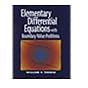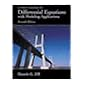Normal view

# A first course in differential equations with modeling applications / Dennis G. Zill.

Material type:TextLanguage: English Publisher: Pacific Grove, CA : Brooks/Cole Thomson Learning, c2001Edition: 7th edDescription: xiii, (various pagings) : ill. (some col.) ; 27 cmISBN: 0534379990 (alk. paper); 9780534379995DDC classification: 515.35 LOC classification: QA372 | .Z54 2001bOnline resources: WorldCat details
Contents:
Table of contents Preface -- Acknowledgments -- 1. Introduction to differential equations -- 1.1. Definitions and terminology -- 1.2. Initial-value problems -- 1.3. Differential equations as mathematical models -- Chapter 1 in review -- 2. First-order differential equations -- 2.1. Solution curves without the solution -- 2.2. Separable variables -- 2.3. Linear equations -- 2.4. Exact equations -- 2.5. Solutions by substitutions -- 2.6. A numerical solution -- Chapter 2 in review -- 3. Modeling with first-order differential equations -- 3.1. Linear equations -- 3.2. Nonlinear equations -- 3.3. Systems of linear and nonlinear differential equations -- Chapter 3 in review -- Project module : harvesting of renewable natural resources / Gilbert N. Lewis -- 4. Higher-order differential equations -- 4.1. Preliminary theory : linear equations -- 4.1.1. Initial-value and boundary-value problems -- 4.1.2. Homogeneous equations -- 4.1.3. Nonhomogeneous equations -- 4.2. Reduction of order -- 4.3. Homogeneous linear equations with constant coefficients -- 4.4. Undetermined coefficients--superposition approach -- 4.5. Undetermined coefficients--annihilator approach -- 4.6. Variation of parameters -- 4.7. Cauchy-Euler equation -- 4.8. Solving systems of linear equations by elimination -- 4.9. Nonlinear equations -- Chapter 4 in review -- 5. Modeling with higher-order differential equations -- 5.1. Linear equations : initial-value problems -- 5.1.1. Spring/mass systems : free undamped motion -- 5.1.2. Spring/mass systems : free damped motion -- 5.1.3. Spring/mass systems : driven motion -- 5.1.4. Series circuit analogue -- 5.2. Linear equations : boundary-value problems -- 5.3. Nonlinear equations -- Chapter 5 in review -- Project module : the collapse of the Tacoma Narrows suspension bridge / Gilbert N. Lewis -- 6. Series solutions of linear equations -- 6.1. Solutions about ordinary points -- 6.1.1. Review of power series -- 6.1.2. Power series solutions -- 6.2. Solutions about singular points -- 6.3. Two special equations -- Chapter 6 in review -- 7. The Laplace transform -- 7.1. Definition of the Laplace transform -- 7.2. Inverse transform and transforms of derivatives -- 7.3. Translation theorems -- 7.3.1. Translation on the s-axis -- 7.3.2. Translation on the t-axis -- 7.4. Additional operational properties -- 7.5. Dirac Delta function -- 7.6. Systems of linear equations -- Chapter 7 in review -- 8. Systems of linear first-order differential equations -- 8.1. Preliminary theory -- 8.2. Homogeneous linear systems with constant coefficients -- 8.2.1. Distinct real eigenvalues -- 8.2.2. Repeated eigenvalues -- 8.2.3. Complex eigenvalues -- 8.3. Variation of parameters -- 8.4. Matrix exponential -- Chapter 8 in review -- Project module : earthquake shaking of multistory buildings / Gilbert N. Lewis -- 9. Numerical solutions of ordinary differential equations -- 9.1. Euler methods and error analysis -- 9.2. Runge-Kutta methods -- 9.3. Multistep methods -- 9.4. Higher-order equations and systems -- 9.5. Second-order boundary-value problems -- Chapter 9 in review -- Appendixes -- I. Gamma functions -- II. Introduction to matrices -- III. Laplace transforms -- Selected answers for odd-numbered problems -- Index.
Summary: Master differential equations and succeed in your course with A FIRST COURSE IN DIFFERENTIAL EQUATIONS WITH MODELING APPLICATIONS with accompanying CD-ROM and technology! Straightfoward and readable, this mathematics text provides you with tools such as examples, explanations, definitions, and applications designed to help you succeed. The accompanying DE Tools CD-ROM makes helps you master difficult concepts Read more...
Tags from this library: No tags from this library for this title.Average rating: 0.0 (0 votes)
Item type Current location Collection Call number Copy number Status Date due Barcode Item holdsText
Reserve Section
Non-fiction 515.35 ZIF 2001 (Browse shelf) C-1 Not For Loan 12662Text
Circulation Section
Non-fiction 515.35 ZIF 2001 (Browse shelf) C-2 Available 12663Text
Circulation Section
Non-fiction 515.35 ZIF 2001 (Browse shelf) C-3 Available 12664Text
Circulation Section
Non-fiction 515.35 ZIF 2001 (Browse shelf) C-4 Available 12665Text
Circulation Section
Non-fiction 515.35 ZIF 2001 (Browse shelf) C-5 Available 12666
Total holds: 0
##### Browsing EWU Library shelves, Shelving location: Reserve Section Close shelf browserNo cover image available 515.35 SID 1991 Differential equations, with applications and historical notes / 515.35 SID 1991 Differential equations, with applications and historical notes / 515.35 TRE 2001 Elementary differential equations with boundary value problems / 515.35 ZIF 2001 A first course in differential equations with modeling applications / 515.35 ZIF 2005 A first course in differential equations with modeling applications / 515.35 ZIF 2005 A first course in differential equations with modeling applications / 515.352 CHD 2014 Differential Equations with Applications/

Rev. ed. of: A first course in differential equations. 5th ed. c1993.

Includes index.

Acknowledgments --
1. Introduction to differential equations --
1.1. Definitions and terminology --
1.2. Initial-value problems --
1.3. Differential equations as mathematical models --
Chapter 1 in review --
2. First-order differential equations --
2.1. Solution curves without the solution --
2.2. Separable variables --
2.3. Linear equations --
2.4. Exact equations --
2.5. Solutions by substitutions --
2.6. A numerical solution --
Chapter 2 in review --
3. Modeling with first-order differential equations --
3.1. Linear equations --
3.2. Nonlinear equations --
3.3. Systems of linear and nonlinear differential equations --
Chapter 3 in review --
Project module : harvesting of renewable natural resources / Gilbert N. Lewis --
4. Higher-order differential equations --
4.1. Preliminary theory : linear equations --
4.1.1. Initial-value and boundary-value problems --
4.1.2. Homogeneous equations --
4.1.3. Nonhomogeneous equations --
4.2. Reduction of order --
4.3. Homogeneous linear equations with constant coefficients --
4.4. Undetermined coefficients--superposition approach --
4.5. Undetermined coefficients--annihilator approach --
4.6. Variation of parameters --
4.7. Cauchy-Euler equation --
4.8. Solving systems of linear equations by elimination --
4.9. Nonlinear equations --
Chapter 4 in review --
5. Modeling with higher-order differential equations --
5.1. Linear equations : initial-value problems --
5.1.1. Spring/mass systems : free undamped motion --
5.1.2. Spring/mass systems : free damped motion --
5.1.3. Spring/mass systems : driven motion --
5.1.4. Series circuit analogue --
5.2. Linear equations : boundary-value problems --
5.3. Nonlinear equations --
Chapter 5 in review --
Project module : the collapse of the Tacoma Narrows suspension bridge / Gilbert N. Lewis --
6. Series solutions of linear equations --
6.1. Solutions about ordinary points --
6.1.1. Review of power series --
6.1.2. Power series solutions --
6.2. Solutions about singular points --
6.3. Two special equations --
Chapter 6 in review --
7. The Laplace transform --
7.1. Definition of the Laplace transform --
7.2. Inverse transform and transforms of derivatives --
7.3. Translation theorems --
7.3.1. Translation on the s-axis --
7.3.2. Translation on the t-axis --
7.5. Dirac Delta function --
7.6. Systems of linear equations --
Chapter 7 in review --
8. Systems of linear first-order differential equations --
8.1. Preliminary theory --
8.2. Homogeneous linear systems with constant coefficients --
8.2.1. Distinct real eigenvalues --
8.2.2. Repeated eigenvalues --
8.2.3. Complex eigenvalues --
8.3. Variation of parameters --
8.4. Matrix exponential --
Chapter 8 in review --
Project module : earthquake shaking of multistory buildings / Gilbert N. Lewis --
9. Numerical solutions of ordinary differential equations --
9.1. Euler methods and error analysis --
9.2. Runge-Kutta methods --
9.3. Multistep methods --
9.4. Higher-order equations and systems --
9.5. Second-order boundary-value problems --
Chapter 9 in review --
Appendixes --
I. Gamma functions --
II. Introduction to matrices --
III. Laplace transforms --
Selected answers for odd-numbered problems --
Index.

Master differential equations and succeed in your course with A FIRST COURSE IN DIFFERENTIAL EQUATIONS WITH MODELING APPLICATIONS with accompanying CD-ROM and technology! Straightfoward and readable, this mathematics text provides you with tools such as examples, explanations, definitions, and applications designed to help you succeed. The accompanying DE Tools CD-ROM makes helps you master difficult concepts Read more...

Computer Science & Engineering Mathematics and Physical Sciences

There are no comments for this item.